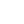# C#: How to Get Enum Member Name with the Related Int Value

By Henri ParviainenIf you want to get access to a name value in a specific enum member in C#, there are actually multiple ways to achieve this. In this article, we will introduce two ways to attain this.

Let's say we have the following enum for an order status in an online store.

``````public enum OrderStatus
{
Processing = 2,
Completed = 3,
Cancelled = 4
}``````

Now, let's say that the `OrderStatus` for some order is `1` and you would like the customer to see `Received` instead of `1`.

Here are two solutions for getting the name value out of enum by using the int constant that we have access to (`1`).

## Solution 1 - Using Explicit Casting with ToString()

The first solution is the most simple one.

We can get access to any related string value of an enum member's int by simply calling `ToString()` to an explicitly casted instance of the represented int value.

``````var value = 1;

string enumName = ((OrderStatus)value).ToString();

## Solution 2 - Using Enum.GetName Method

Another option is to use `Enum.GetName(Type, object)`.

`Type` stands for the enum type we have declared.

`object` stands for the value of a particular enumerated constant.

``````var value = 1;

string enumName = Enum.GetName(typeof(OrderStatus), value);

Learn more about `Enum.GetName` method in the Microsoft .NET docs.

## Conclusion

Although we get equal results with both methods introduced above when we use valid data, the methods work differently if they are given invalid data as an input.

Let's imagine our invalid data is `5`. It is not part of any enum member and thus it is invalid.

If we use the first method with this invalid data, the output will be `'5'` since it will just take the int 5 and turn it into a string.

If we use the latter method we will get `null` as the output.

For this reason, I would recommend using the latter solution with Enum.GetName method when there is a risk of getting invalid data since `null` would be a more desirable outcome than the given input turned into a string.